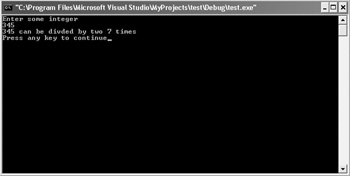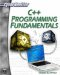# Recursion

Recursion is a relatively common programming technique. You are bound to encounter it frequently. It is actually a relatively simple concept. Recursion refers to a function that calls itself. This is frequently used in a variety of algorithms, including factoring algorithms. The function continuously calls itself until a final point is reached, such as the number being completely factored, then the function quits calling itself.

### Example 13.5

Step 1: Enter the following code into a text editor and save it as 13.05.cpp.

`#include <iostream> using namespace std; int findmultiplesoftwo(int); int main() { int input,answer; cout << "Enter some integer \n"; cin >> input; answer =findmultiplesoftwo(input); cout << input << " can be divided by two "; cout << answer << " times \n"; return 0; }// end of main int findmultiplesoftwo(int number) { // This function finds out how many times // two will go into whatever number is passed to // it.  It does this via recursion. static int count; number = number / 2; count++; //increment the counter if (number > 2) {       findmultiplesoftwo(number); } else {       return count; }    }`

Step 2: Compile and execute the code. You should see something like what is shown in Figure 13.6.Figure 13.6: Recursion.

You can see here that this function calls itself repeatedly. Recursion is often used in more advanced sorting algorithms. If you continue your studies of algorithms, you will undoubtedly encounter recursion. You also saw a new C++ command used here. It was the word static. When a variable is defined locally, within a function, as soon as you exit the function, or call it again, that variable gets recreated. Its value is not retained between iterations of a function. If you put the word static before the variable declaration, you are instructing the compiler to retain that variable’s value between iterations of the function.

Thus, a recursive function is one that calls itself. A function that does this is said to be recursive. It is important that you be familiar with these two terms, as you will probably encounter them frequently in various programming examples.C++ Programming Fundamentals (Cyberrookies)
ISBN: 1584502371
EAN: 2147483647
Year: 2005
Pages: 197
Authors: Chuck Easttom